# 3D Visualization of the rays of E6 & E7 in Kochen-specker theory by Ruuge & Waegell/Aravind

In several papers on BKS proofs, Arthur Ruuge’s “Exceptional and Non-Crystallograpic Root Systems and the Kochen-Specker Theorem” https://arxiv.org/abs/0906.2696v1 and Mordecai Waegell & P.K. Aravind’s “Parity proofs of the Kochen-Specker theorem based on the Lie algebra E8” https://arxiv.org/abs/1502.04350v2, in addition to E8, E6 and E7 is studied. Using the visualization developed for my recent paper and prior papers, I present here the related visualizations for E6 and E7 as discussed in those papers.The 72 E6 vertices derived from E8 and projected to 3D using the “E8->H4” basis vectors. The 15*72=1080 edges are shown in the upper left, the 36 anti-podal rays are shown in the upper right along with the hull group vertex counts and norm distance. The bottom image shows the 4 hulls – yellow Icosahedron (12), cyan dodecahedron (20) and the orange/pink pentagonal prisms (40)).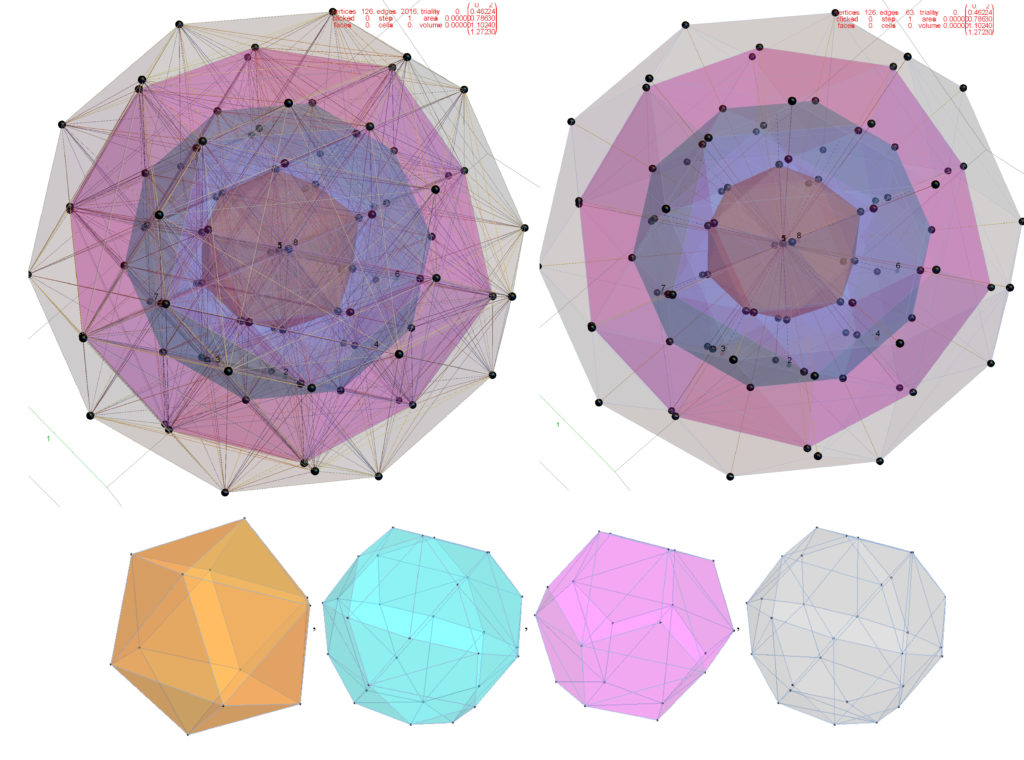The 126 E7 vertices derived from E8 and projected to 3D using the “E8->H4” basis vectors. The 16*126=2016 edges are shown in the upper left, the 63 anti-podal rays are shown in the upper right along with the hull group vertex counts and norm distance. The bottom image shows the 4 hulls – orange 2 overlapping Icosahedrons (24), pink2 overlapping dodecahedron (40), and cyan & gray icosidodecahedrons(60) with 2 vertices at the origin.

# My Latest paper published on Vixra – 3D Polytope Hulls of E8 4_21, 2_41, and 1_42

https://vixra.org/pdf/2005.0200v1.pdf

or also available directly from this website:

https://theoryofeverything.org/TOE/JGM/3D_Polytope_Hulls_of_E8-421-241-142.pdf

Using rows 2 through 4 of a unimodular 8x8 rotation matrix, the vertices of E8 421, 241, and 142 are projected to 3D and then gathered & tallied into groups by the norm of their projected locations. The resulting Platonic and Archimedean solid 3D structures are then used to study E8’s relationship to other research areas, such as sphere packings in Grassmannian spaces, using E8 Eisenstein Theta Series in recent proofs for optimal 8D and 24D sphere packings, nested lattices, and quantum basis critical parity proofs of the Bell-Kochen-Specker (BKS) theorem.

A few new Figures from the paper.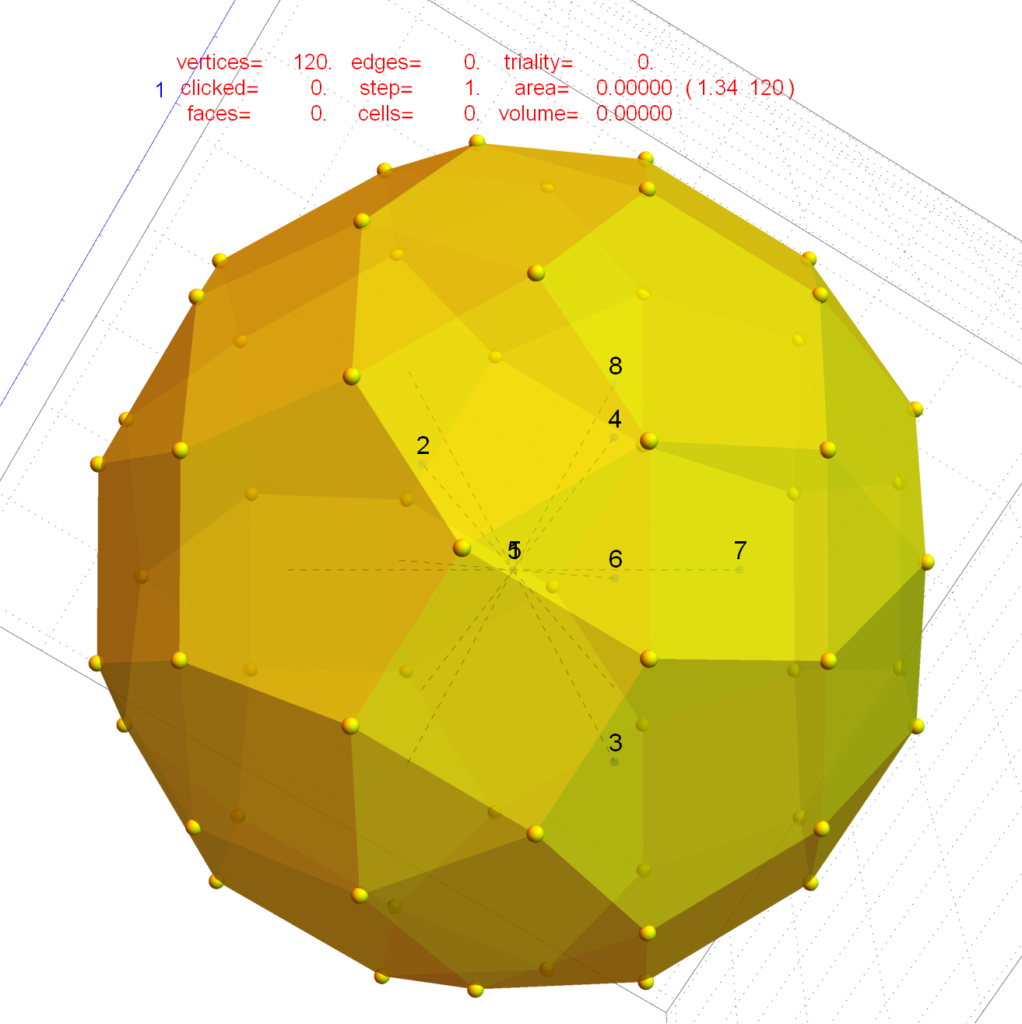FIG. 6: Pair of overlapping rhombicosidodecahedrons from3rd largest hull of the 74 hulls in 142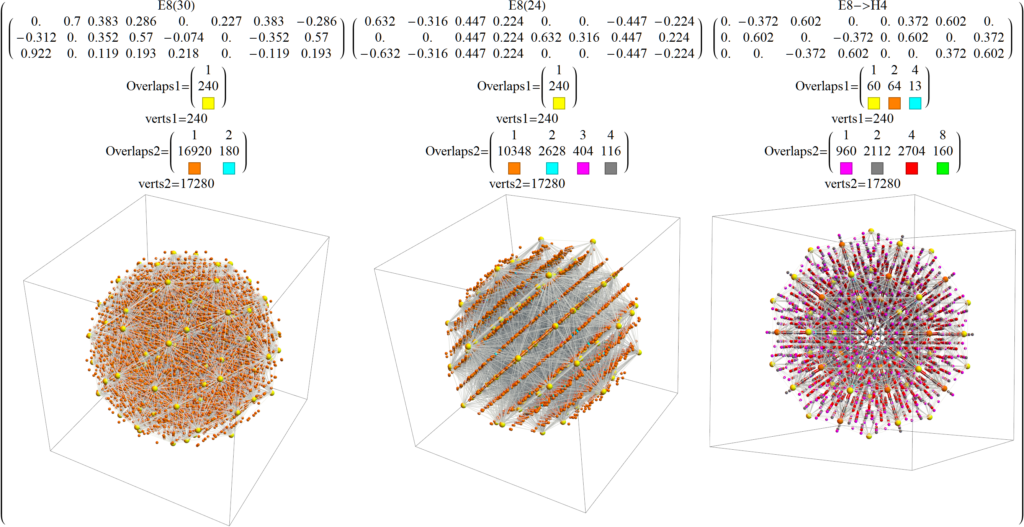FIG. 13: 421 & Polytope projected to various 3D spacesEach 3D projection shown lists the projection name, the nu-meric basis vectors used, and the 421 & 142 overlap color codedvertex groups, and the projection with vertices (larger) &6720 edges and the 142 vertices (smaller)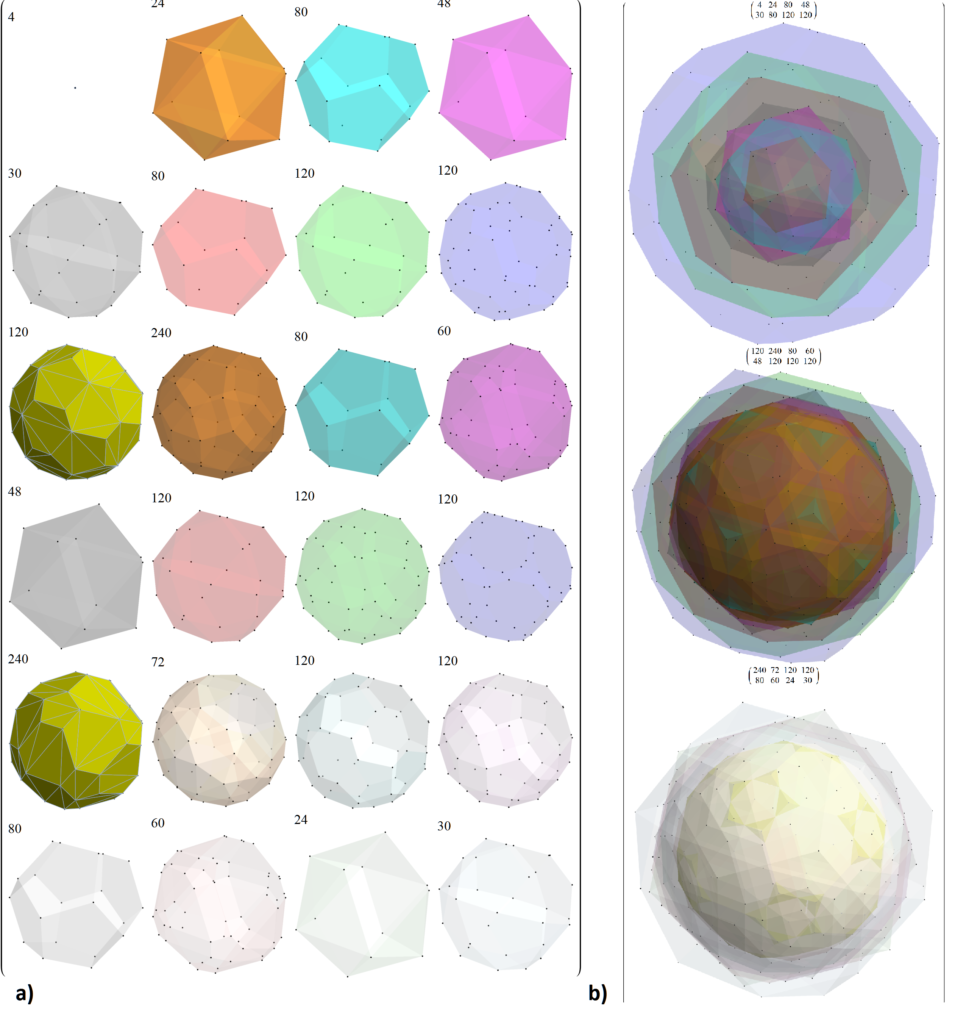FIG. 14: Concentric hulls of 241 in Platonic 3D projectionwith vertex count in each hull and increasing opacity andvaried surface colors.a) 24 individual concentric hulls b) In groups of 8 hulls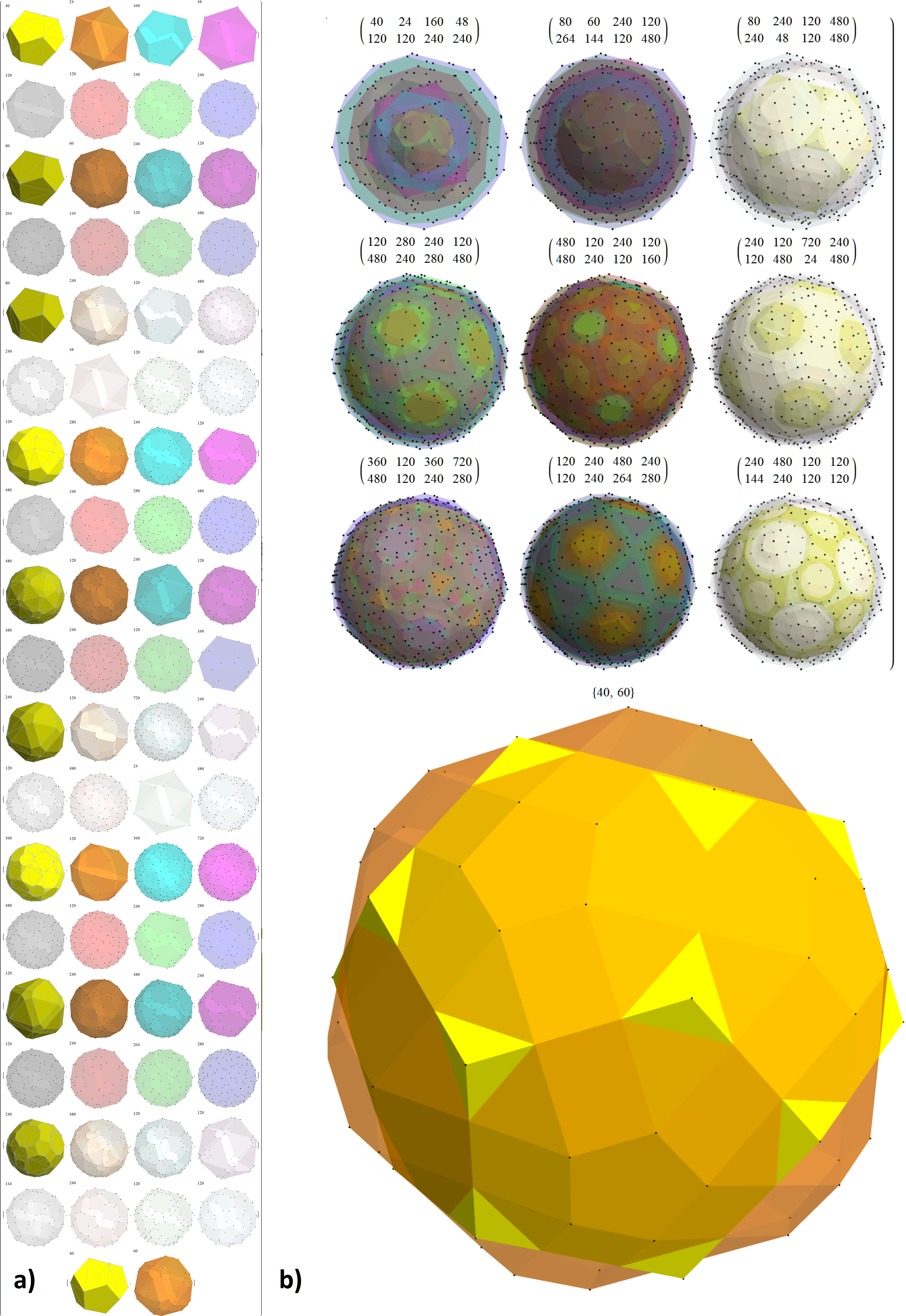FIG. 15: Concentric hulls of 142 in Platonic 3D projectionwith vertex count in each hull and increasing opacity andvaried surface colors.a) 74 individual concentric hullsb) In groups of 8 hulls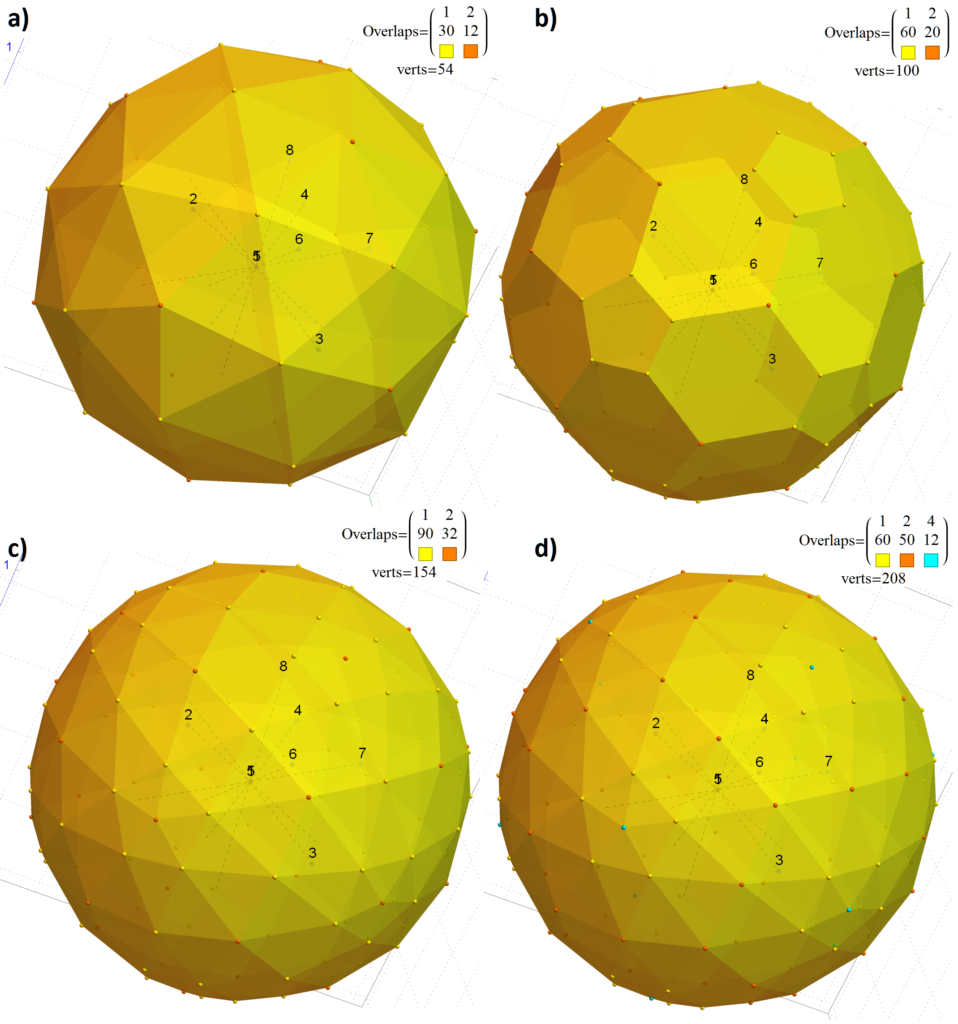FIG. 18: E8’s outer two hulls scaled to unit norms in Platonic3D projection with vertex counts color coded by overlapsa) 54 vertex (42 unique) 421=241 icosidodecahedron (30 yel-low) & two overlapping icosahedrons (12 red) scaled 1.051b) 100 vertex (80 unique) 142 non-uniform rhombicosidodeca-hedron (60 yellow) & two overlapping dodecahedrons (20 red)scaled 1.0092c) 154 vertex (122 unique) combination of a & bd) 208 vertex (122 unique) combination same as c with colorcoded vertex counts for both 421 & 241Note: The internal numbers of the image are the 8 axis (pro-jection basis vectors).

Now for a few new visualizations that are not in the paper…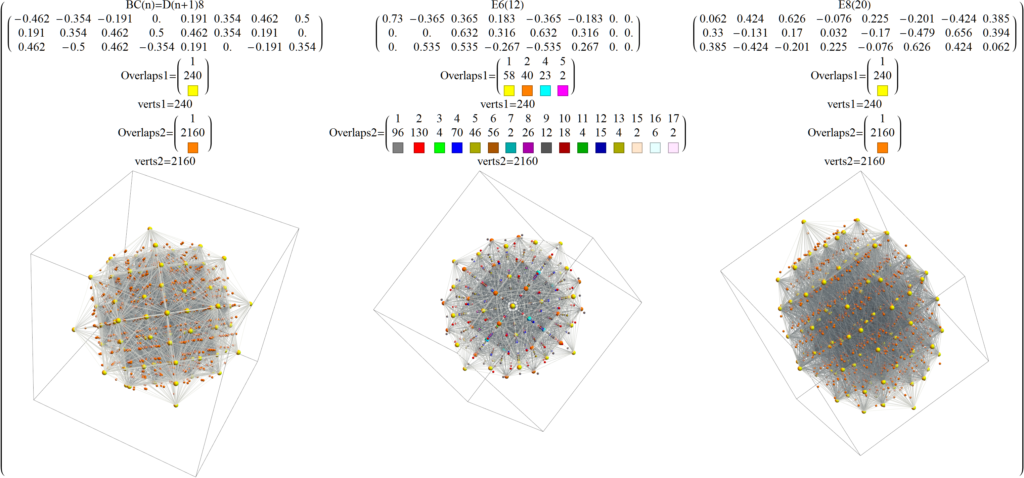Various 3D projections of 2_41Various 3D projections of 4_21

# 3D visualization of E8 1_42 polytope

This is what I expect to be the first ever 3D visualization of the E8 1_42 polytope with 17280 vertices showing concentric hulls of Platonic solid related structures!E8 1_42 polytope with 17280 vertices showing concentric hulls of Platonic solid related structures! Vertex colors represent the overlap counts.Each of 74 concentric hulls based on 3D Norm’d vertex positions (with varying opacity in sets of 8). Vertex counts in each hull listed above.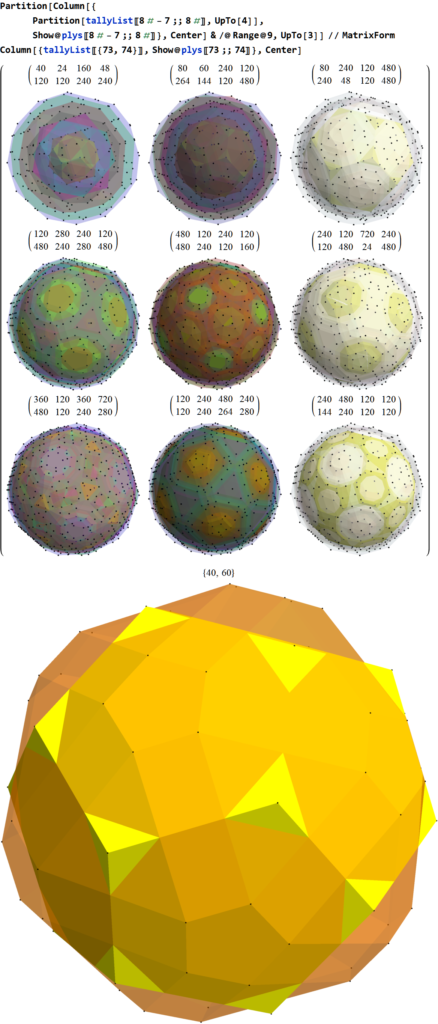9 sets of 8 concentric hulls plus the last 2 outer hulls, Vertex counts in each of 8 hull listed above. Notice this is a combination of two overlapped dodecahedrons (40) and a Nonuniform Rhombicosidodecahedron (60).

For the sake of completeness in visualization, see below for various projections to 2D. Click these links for a higher resolution PNG or the SVG version.

# Nested Lattices of E8 in Complex Projective 4-Space

I read an interesting article about a pattern discovered by Warren D. Smith (discussed at length here):

“The sum of the first three terms in the Eisenstein E_4(q)  Series Integers of the Theta series of the E8 lattice is a perfect fourth power: 1 + 240 + 2160 = 2401 = 7^4”

So I decided to visualize the 2401=1+240+2160 vertex patterns of E8 using my Mathematica codebased toolset based on some previous work I put on my Wikipedia talk page.

The image below represents various projections showing 6720 edges of the 240 E8 vertices, plus a black vertex at the origin, and the 2160 Witting Polytope E8 2 _ 41 vertices using the same projection basis (listed at the top of each image along with the color coded vertex overlaps). Click these links for a higher resolution PNG or the SVG version.

Some of the particular projections of the Witting Polytope may need 8D rotations applied to the basis vectors to find better symmetries with the Gosset, but this is a start using my standard set of projections.

The 240 vertices of the Gosset Polytope are generated using various permutations:

(* E8 4_21 vertices *)
e8421 = Union@Join[
Eperms8@{1, 1, 1, 1, 1, 1, 1, 1}/2,
perms8@{1, 1, 0, 0, 0, 0, 0, 0}];

The 2160 vertices of the Witting Polytope are generated using various permutations:

(* E8 2_41 vertices *)
e8241=Union@Join[
perms8[{1,0,0,0,0,0,0,0}4],
perms8[{1,1,1,1,0,0,0,0}2],
Eperms8[({2,0,0,0,0,0,0,0}+1)]]/4;

Another view shows just the 2160 Witting Polytope vertices. Click these links for a higher resolution PNG or the SVG version.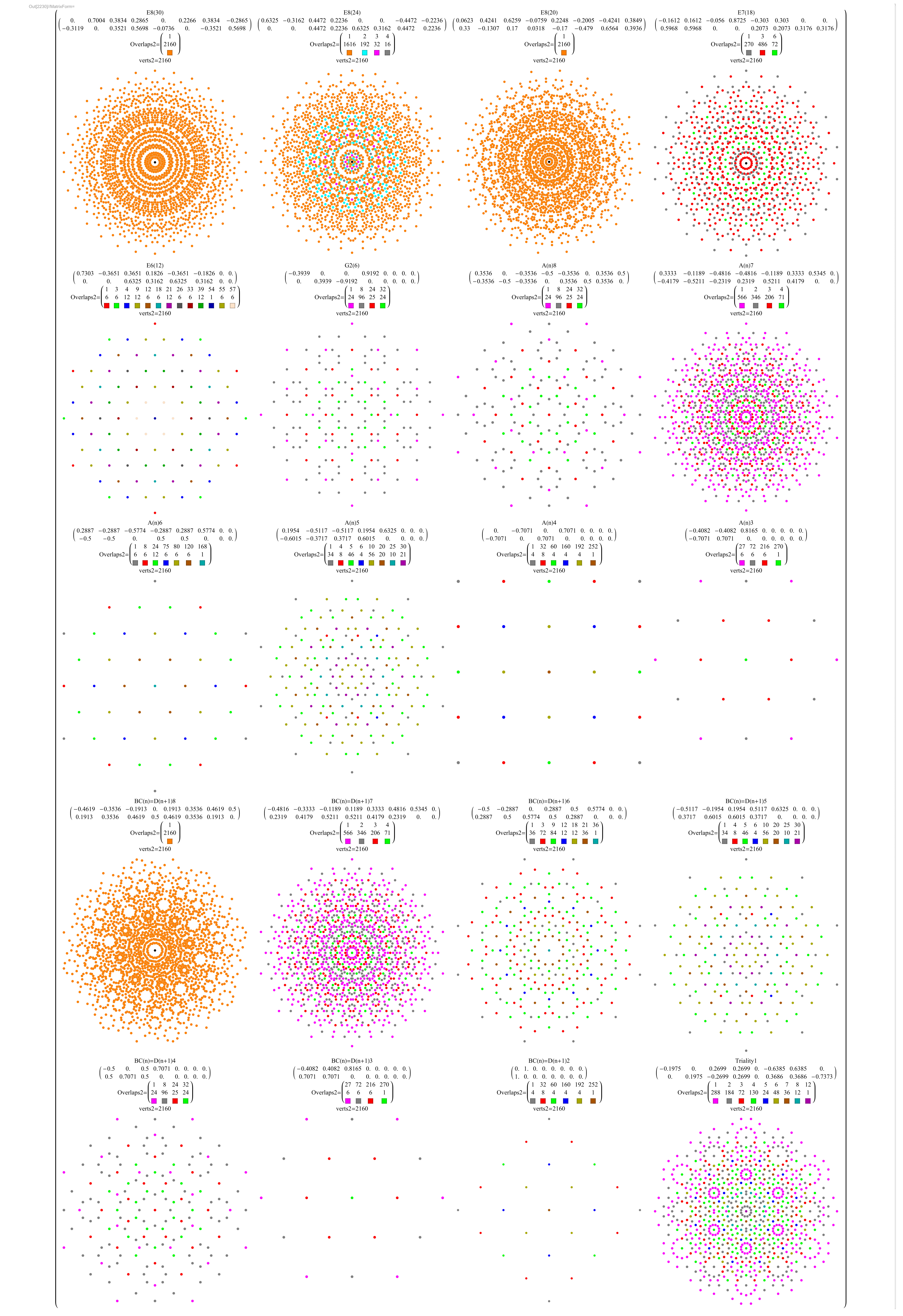2160 Witting Polytope (vertices only)

Another great source of visualizations on E8 and this Witting Polytope is here.

Now visualizing in 3D the structure in 3D using rows 2-4 of the E8->H4 folding matrix, we get:Witting Polytope with 2160 E8 2 _ 41 vertices sorted by Norm distance into 3D Platonic solid related concentric hull structures using rows 2-4 of the E8->H4 folding matrix. The last image shows overlap counts using colored vertices.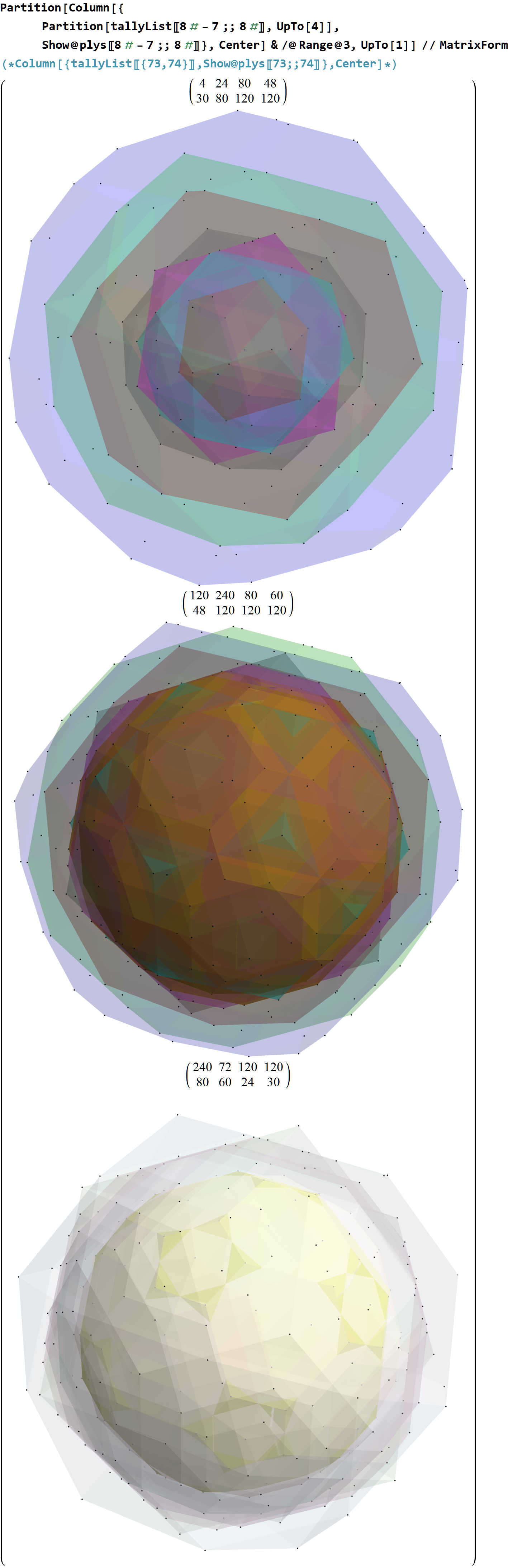Sets of 8 concentric hulls with hull vertex counts above each.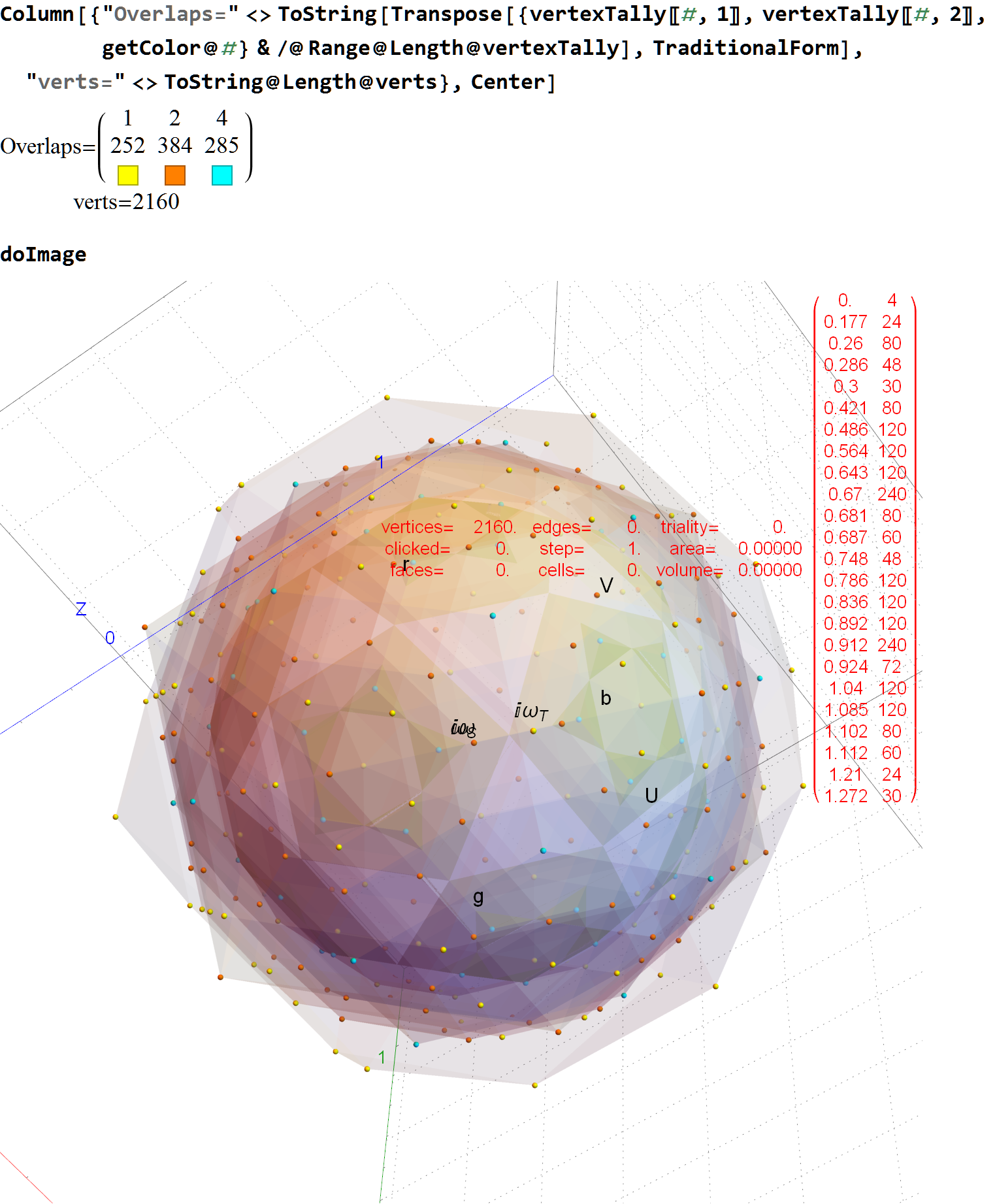Full set of concentric hulls with color coded vertices showing overlap counts. Norm’d vertex groups in red.

# Latest Paper – Unimodular rotation of E8 to H4 600-cells

## Please see my latest paper that describes some advances in understanding the E8 to H4 rotation matrix

https://theoryofeverything.org/TOE/JGM/Unimodular-Rotation-of-E8-to-H4.pdf

Abstract: We introduce a unimodular Determinant=1 8×8 rotation matrix to produce four 4 dimensional copies of H4 600-cells from the 240 vertices of the Split Real Even E8 Lie group. Unimodularity in the rotation matrix provides for the preservation of the 8 dimensional volume after rotation, which is useful in the application of the matrix in various fields, from theoretical particle physics to 3D visualization algorithm optimization.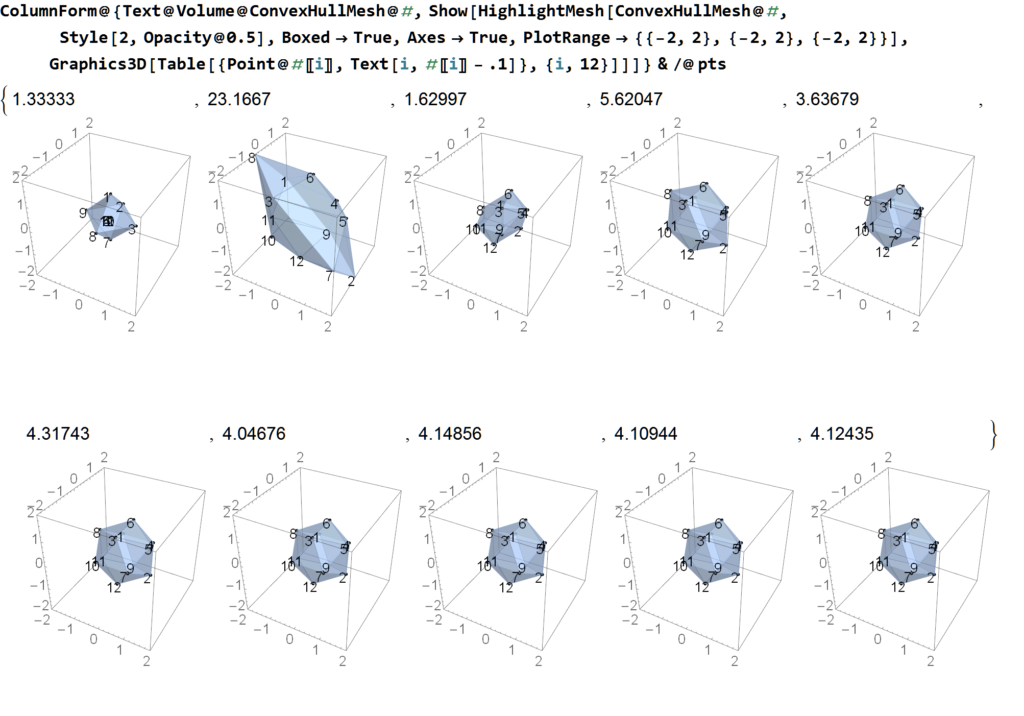Visualization of the Icosahedral points generated recursively…

# Mathematica Analysis of Cohl Furey’s octonion and Clifford group theoretic ℂ⊗O assignments to standard model particles

I’ve read some recent papers by Cohl Furey and was intrigued by the potential relationship between the octonion and Clifford group theoretic assignments to standard model particles. Since I have developed an extensive Mathematica notebook to perform symbolic analysis using these structures (derived primarily for E8 Lie group work), I decided to follow her suggestion… “The reader is encouraged to check that C⊗O forms the 64-complex-dimensional Clifford algebra Cl(6), generated by the set {i e1,…i e6} acting on f”.

So to that end, I created the this .pdf with some preliminary results of that analysis. I found a few minor issues, but so far the model seems to symbolically compute consistently. Very cool!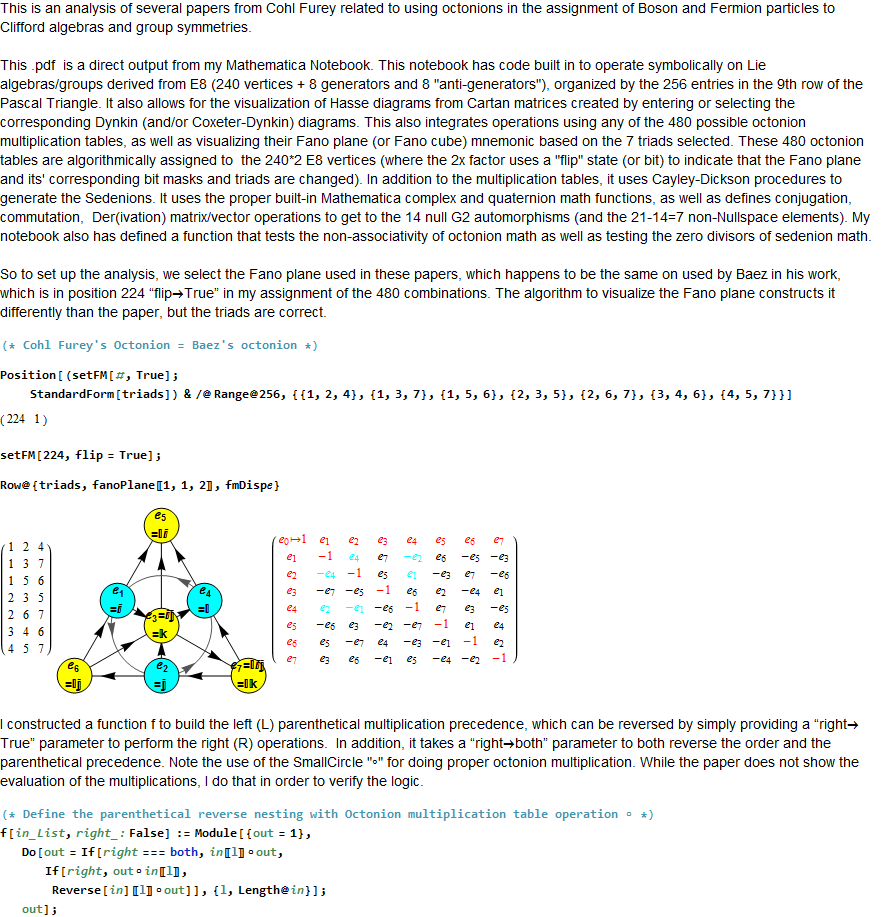# Latest paper: Even FibBinary Numbers and the Golden Ratio

Please see my latest paper here.

It adds information related to my previous blog post here.

Abstract: Previously, a determination of the relationship between the Natural numbers (N) and the n’th odd fibbinary number has been made using a relationship with the Golden ratio phi=(Sqrt+1}/2 and tau=1/phi. Specifically, if the n’th odd fibbinary equates to the j’th N, then j=Floor[n phi^2 – 1]. This note documents the completion of the relationship for the even fibbinary numbers, such that if the n’th even fibbinary equates to the j’th N, then j=Floor[n phi]-1, starting at j=0 for n=1. Alternatively, starting at j=2 for n=1, then j=Floor[n phi + tau].

Title:Even FibBinary Numbers and the Golden Ratio
Authors: J Gregory Moxness
Comments: Dec 31 2018, 5 pages, 4 Figures.
Subjects: Combinatorics

Below is a listing of the N integers represented by their 38 odd FibBinaries, along with the binary and Gray code Compositions.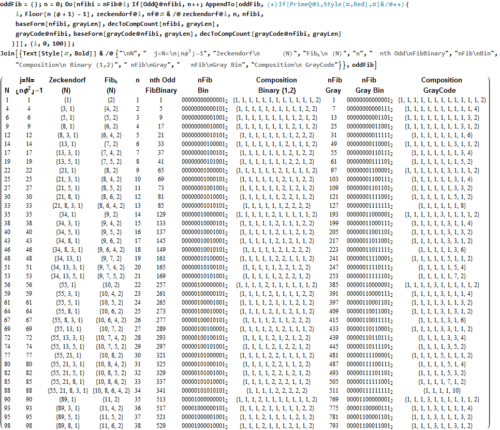Below is a listing of the first 63 N integers represented by their even FibBinaries, along with the binary and Gray code Compositions.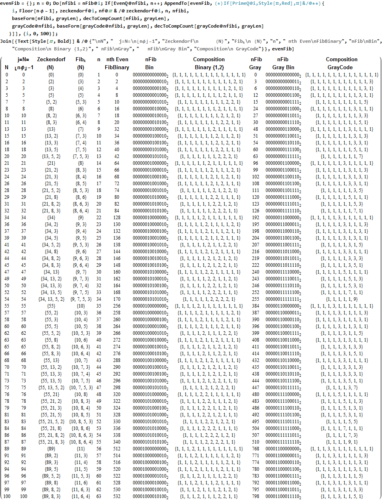Code output examples:Mathematica Code Snippets: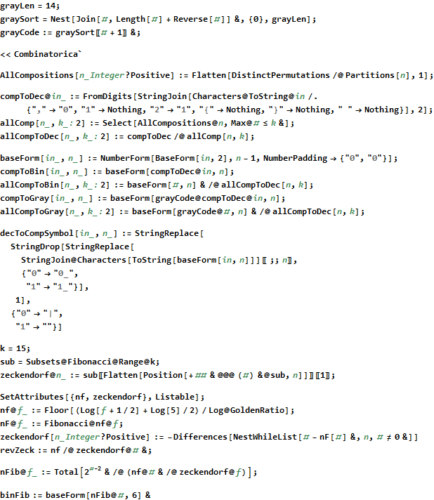# Integer Compositions, Gray Code , and the Fibonacci Sequence

I was prompted by Chris Birke to take a look at this very interesting paper by Linus Lindroos. Chris is working with my E8 to H4 Folding Matrix for optimizing computation using network theory and hyper-dimensional geometric structures.

I was able to validate the paper with some quick Mathematica code.

Below is the code that sets up the generation of the tables, including various binary, numeric, and symbolic (graph) Compositions.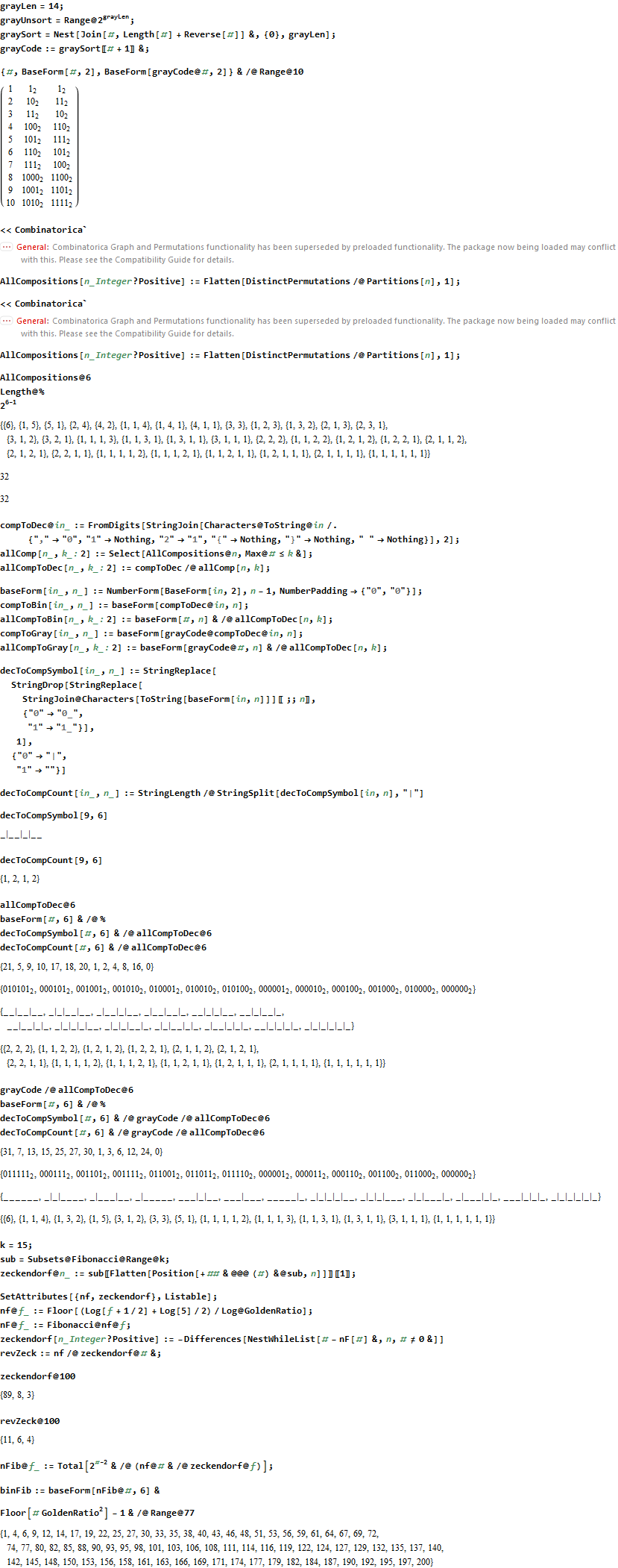Notice the last statement (above) lists the odd FibBinaries (up to N=200) as related to the Floor of the n*Phi^2-1, with n=1 to 77.

Below is a listing of the first 100 N integers represented by their odd and even FibBinaries, along with the binary and Gray code Compositions.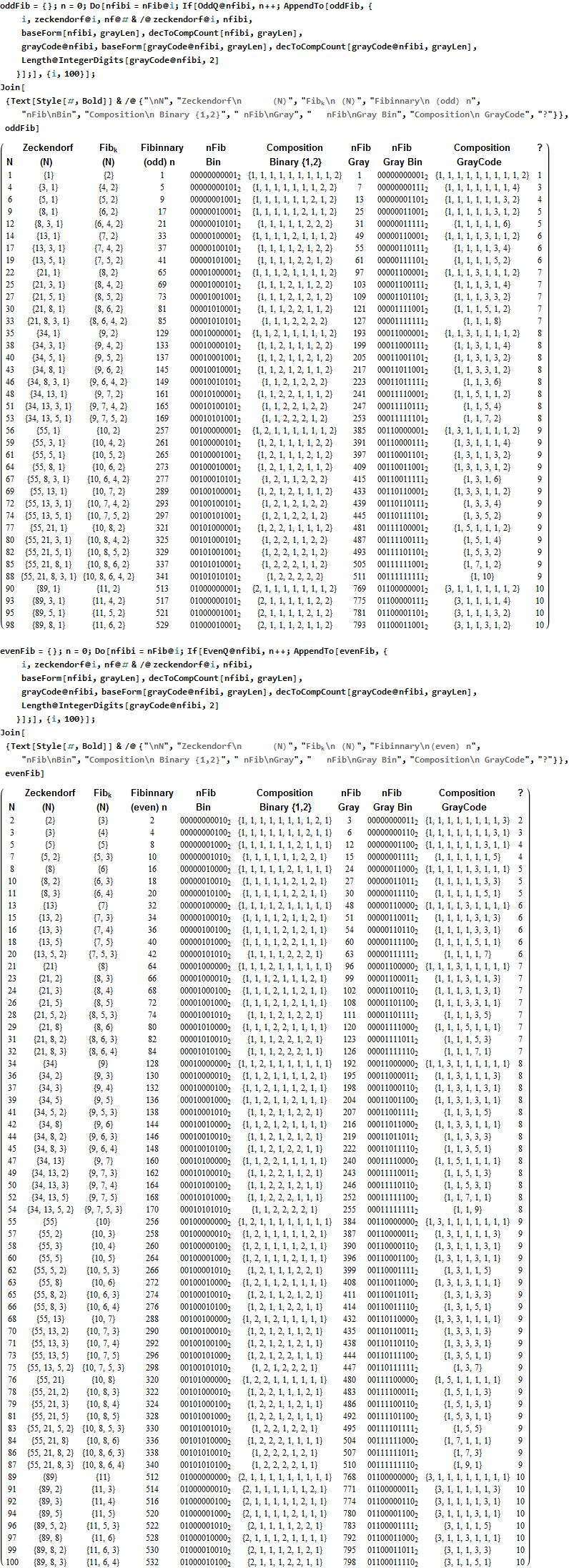# Mapping the fourfold H4 600-cells emerging from E8: A mathematical and visual study

My latest paper is now available here or on Vixra.

{abstract}
It is widely known that the E8 polytope can be folded into two Golden Ratio (Φ) scaled copies of the 4 dimensional (4D) 120 vertex 720 edge H4 600-cell. While folding an 8D object into a 4D one is done by applying the dot product of each vertex to a 4×8 folding matrix, we use an 8×8 rotation matrix to produce four 4D copies of H4 600-cells, with the original two left side scaled 4D copies related to the two right side 4D copies in a very specific way. This paper will describe and visualize in detail the specific symmetry relationships which emerge from that rotation of E8 and the emergent fourfold copies of H4. It will also introduce a projection basis using the Icosahedron found within the 8×8 rotation matrix. It will complete the detail for constructing E8 from the 3D Platonic solids, Icosians, and the 4D H4 600-cell. Eight pairs of Φ scaled concentric Platonic solids are identified directly using the sorted and grouped 3D projected vertex norms present within E8. Finally, we will show the relationship of the Beordijk-Coxeter Tetrahelix emerging from the Petrie projection’s concentric rings of 30 vertices of the H4 600-cells.

This paper builds off of some recent work here, and here.

Below are a few figures from that paper.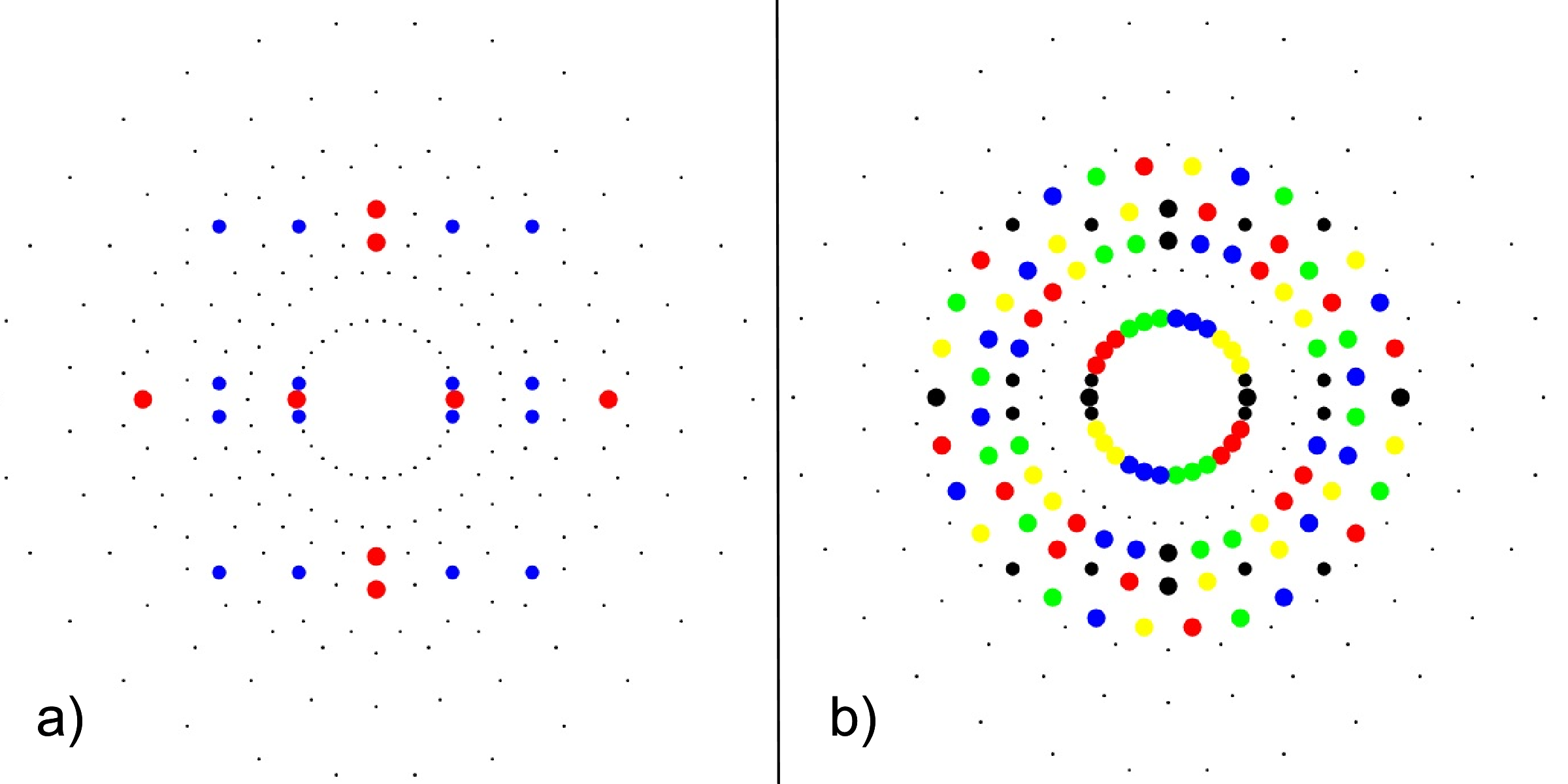FIG. 3: a) 24-cell highlighting the 16-cell (red on-axis vertices) and 8-cell (blue off-axis vertices), b) Snub 24-cell highlighting four pi/5 rotations of the 24-cell (black) in red, green, blue, yellow.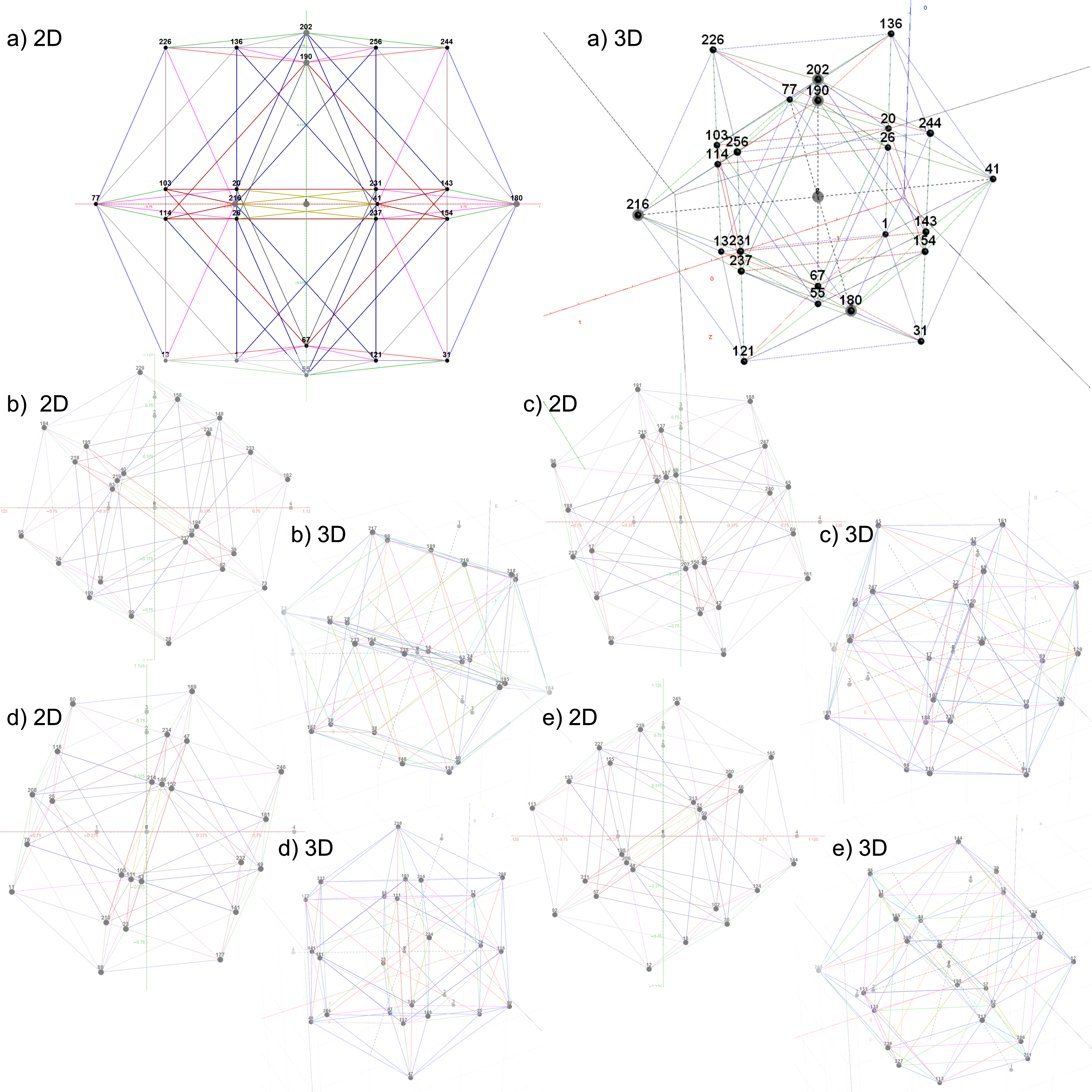FIG. 4: The 5 24-cell decomposition of one 600-cell, each with 2D and 3D vertex numbered projections including edges; a)
24-cell, b-e) Snub 24-cells from four pi/5 rotations of the 24-cell in a)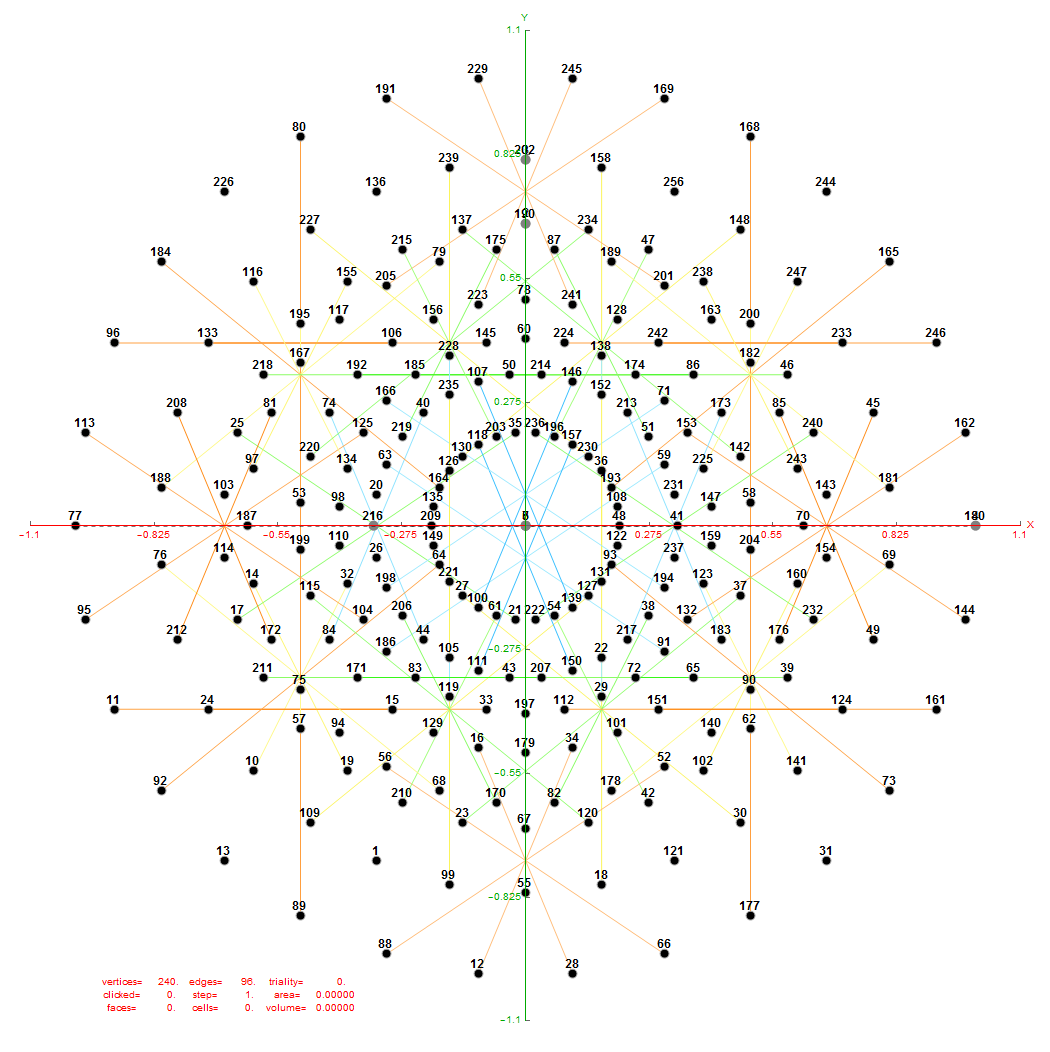FIG. 6: Vertex numbered Petrie projection of the rotated E8, showing the 96 edges of 8D norm l = 2Φ which links the Snub 24-cell H4 and H4Φ (L<->R) vertices (Note: the 8 excluded positive E8 8-Orthoplex (or equivalently, the H4 and H4Φ 4-Orthoplex) “generator vertices” are shown as larger gray labeled axis dots which overlap their darker black E8 vertices)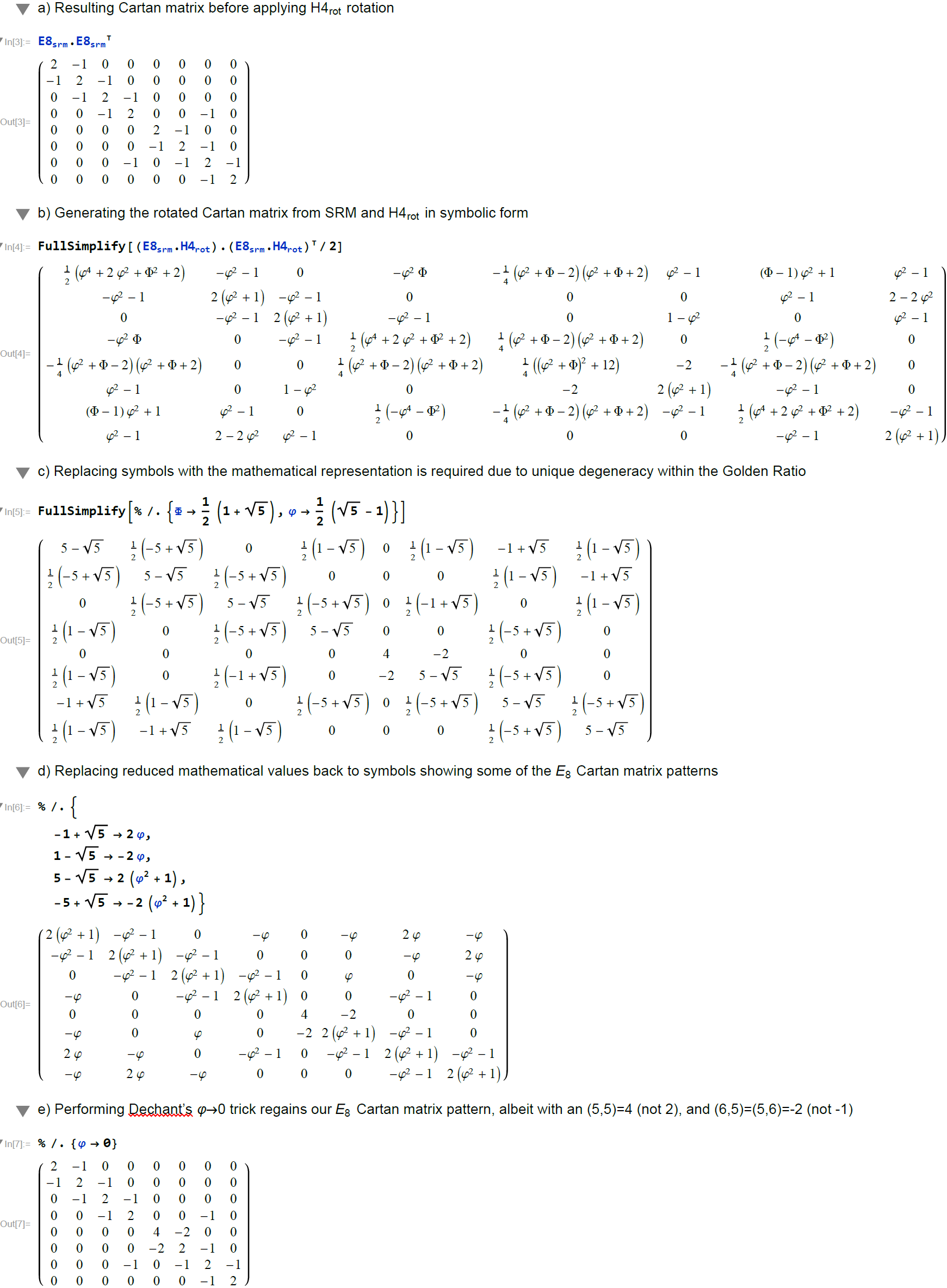FIG. 7: Symbolic analysis using MathematicaTM comparing the Cartan matrix before and after rotating the simple roots matrix (E8srm) used to create it (Note: the resulting Cartan matrix is not precisely that of E8, even after applying the Dechant’s Φ=0 trick)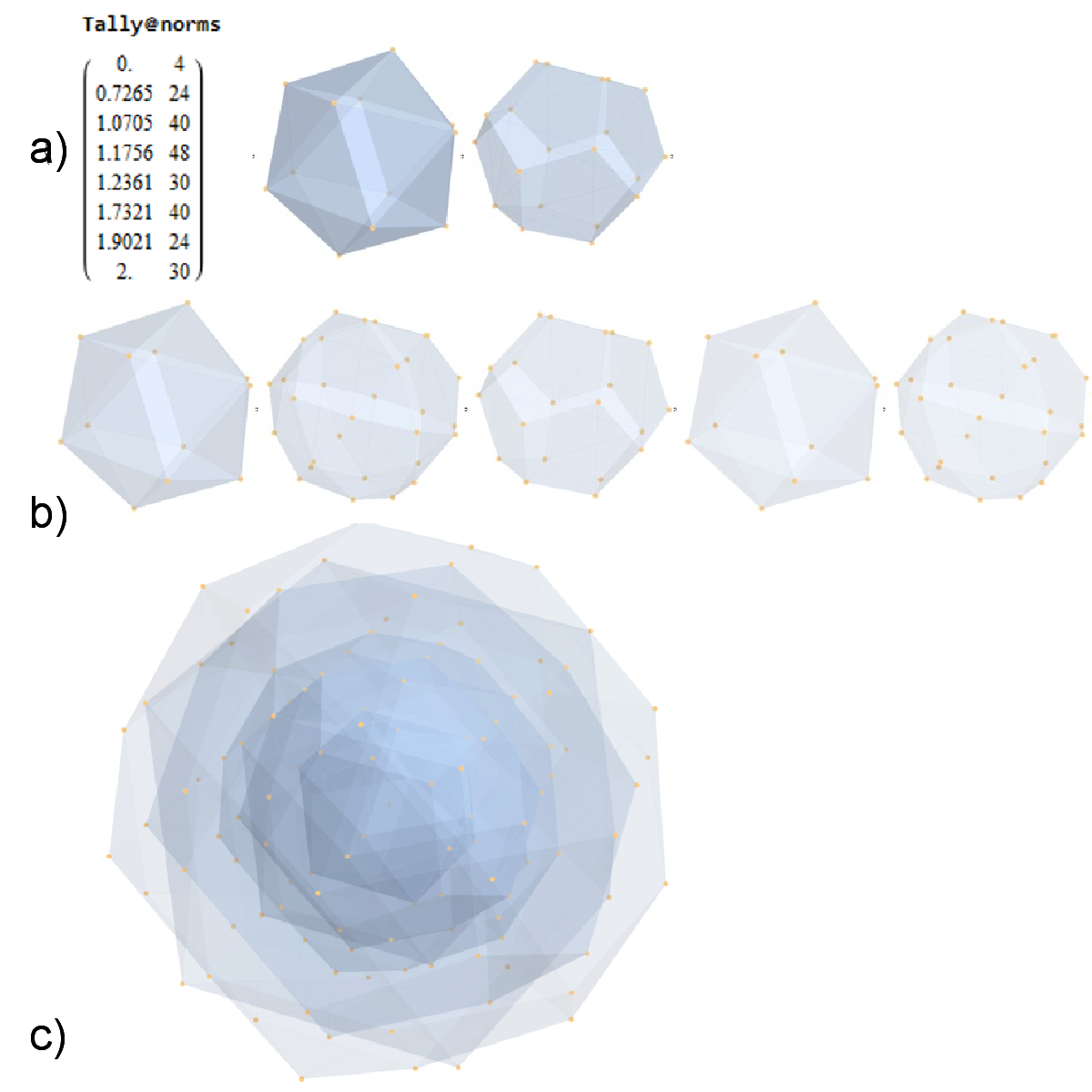FIG. 8: a) Sorted list of the vertex norms with their grouped vertex counts. b) 3D surface models for each of the 7 hulls of vertices c) Combined 3D surface model with increasing transparency for each successive hull.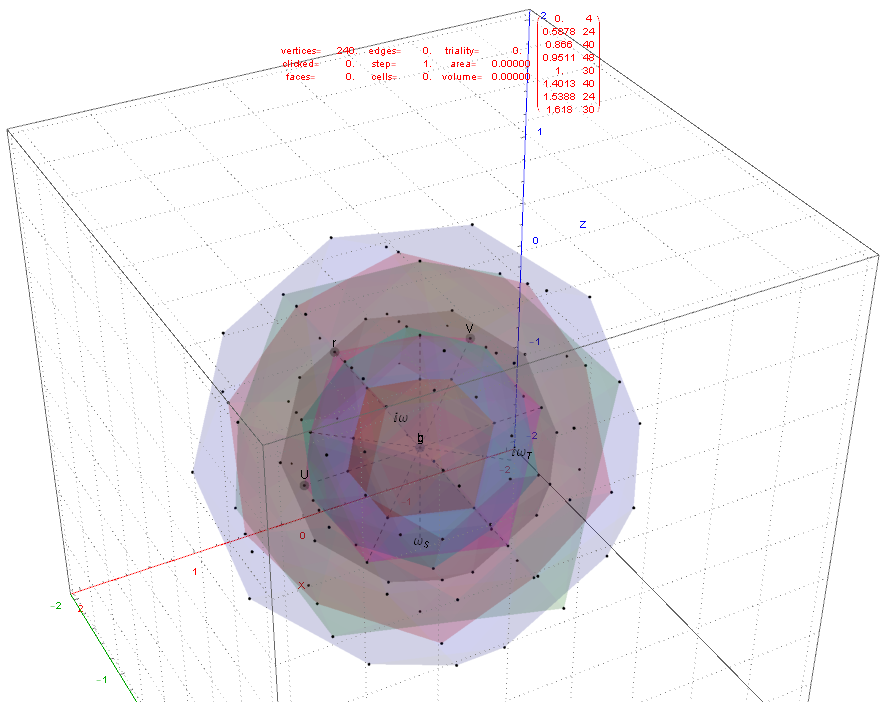FIG. 11: 3D projection using an orthonormal basis on H4 and H4Φ vertices (i.e. the rotated E8)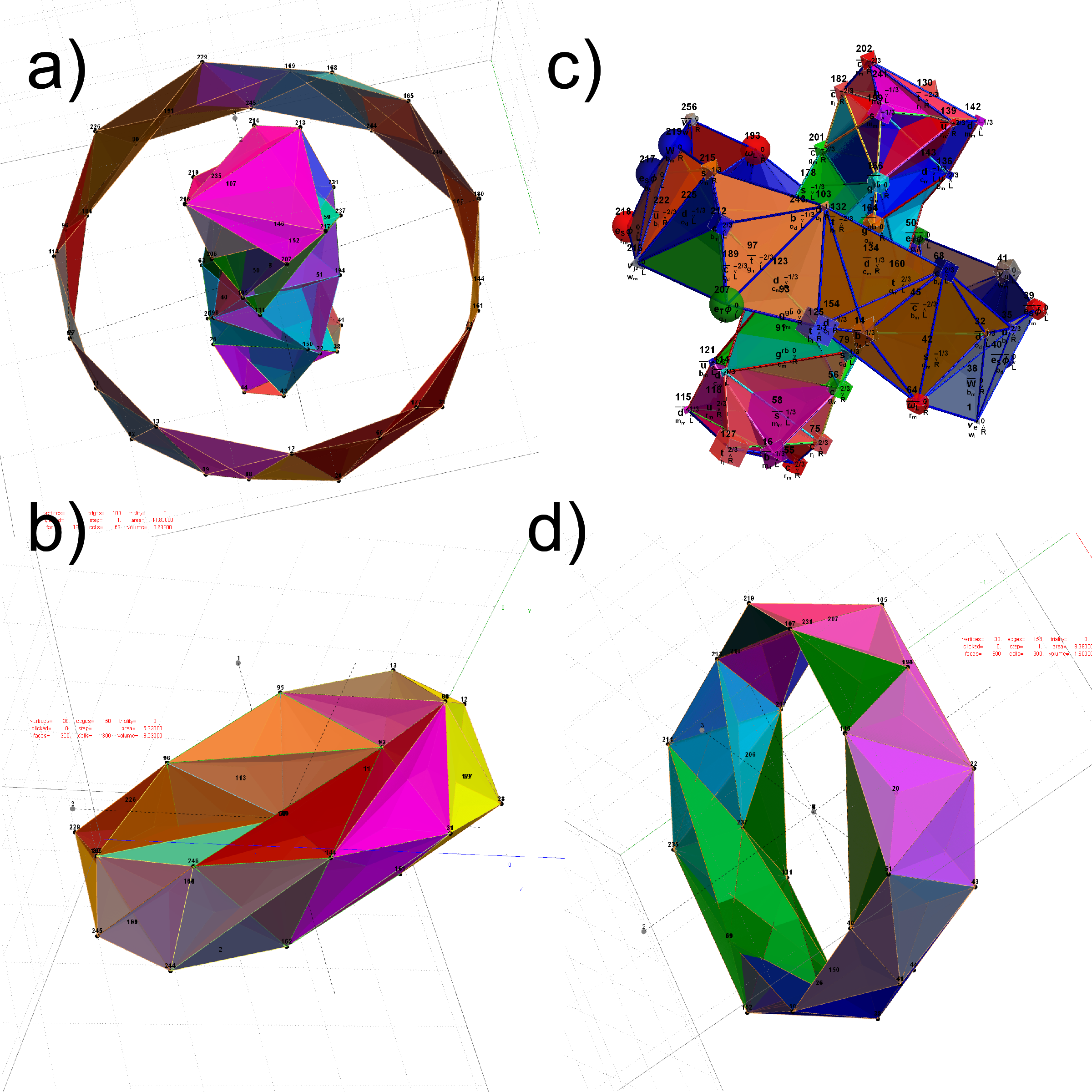FIG. 12: The Beordijk-Coxeter Tetrahelix emerging from the 8 concentric rings of 30 E8 and H4+H4Φ Petrie projection vertices; a) H4 inner and outer ring in 3D, b) with vertex size, shape, color assigned from physics model, c-d) inner and outer ring in orthonormal projection of H4Φ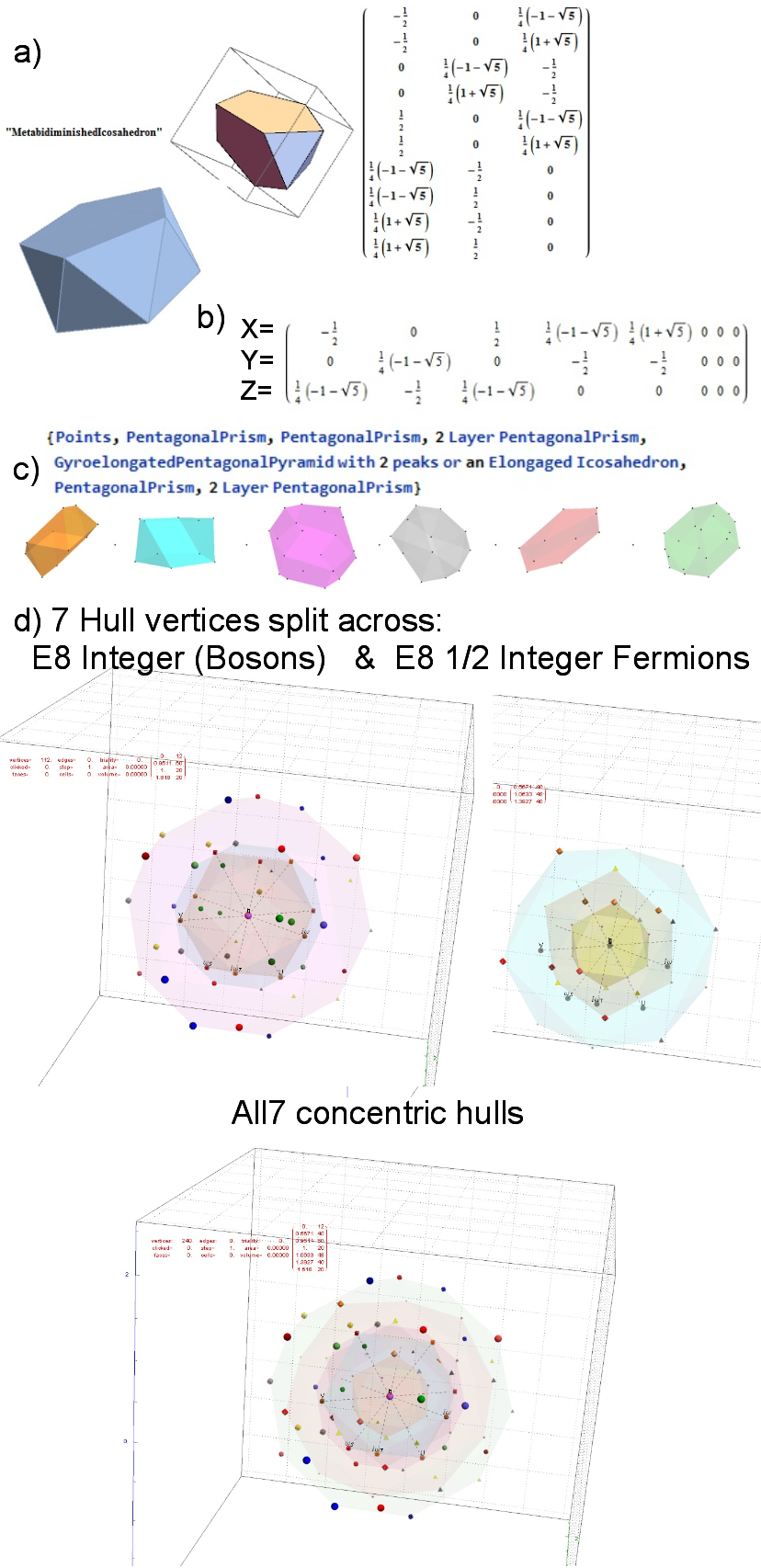FIG. 15: MetabidiminishedIcosahedron (with its negated vertices gives the GyroelongatedPentagonalPyramid) Crystal Projection Prism projecting E8 a) the crystal prism geometry and vertices, b) the selected basis vectors, c) the individual 3D projected concentric hull objects, d) the combined set of concentric objects with progressively increasing transparency and tally of vertex norms.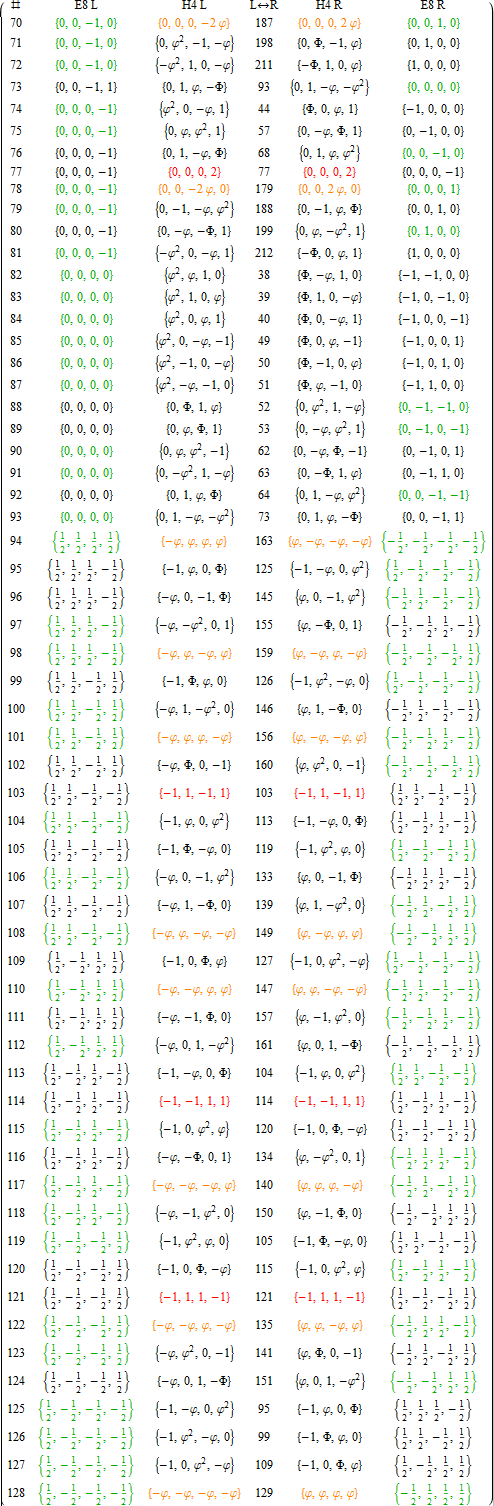APPENDIX A: LIST OF E8 AND ITS ROTATED H4 AND H4Φ VERTICES
This table splits the E8 and its rotated H4 vertices into L/R 4D elements.

1. Table structure
The first column is an SRE E8 vertex index number derived from sorting the E8 vertices by their position based on the 256=2^8 binary pattern from the 9th row of the Pascal triangle (1, 8, 28, 56, 70, 56, 28, 8, 1) and its associated Cl8 Clifford Algebra. This construction is described in more detail in . The odd groups (1,3,5,7,9) with (1,26,70,26,1) elements (respectively) are the 128 1/2 integer vertices. The even groups (2,4,6,8) with (8,56,56,8) elements (respectively) are the 112 integer vertices along with the 16 excluded 8 generator (and 8 anti-generator) vertices (2-9 and 248-255) with permutations of (1; 0; 0; 0; 0; 0; 0; 0) (a.k.a. the 8-Orthoplex), such that they indicate the basis vectors used for projecting the polytope.

Only the first half of the 240 vertices is shown, since the last half is simply the reverse order negation of the vertices
in the first half (e.g. the E8 vertex n=10 has as its’ negation 257-n=247). The middle column labeled L<->R indicates the vertex reference number that contains the same L as the R (and equivalently, the same R as the L, interestingly enough).

2. Table color coding
The E8 L/R columns’ green color-coded elements indicate that the 4D vertex rotates into the smaller H4 600-cell.
Conversely, the E8 L/R columns’ black color-coded elements indicate the 4D vertex rotates into the larger H4Φ
600-cell.

The H4 L/R columns’ red color-coded rows are 24-cell elements that always self-reference and are always members of the scaled up 600-cell H4Φ. The H4 L/R columns’ orange color-coded rows are 24-cell elements that always reference the negated elements in the range of 129-256 and are always members of the smaller 600-cell H4.